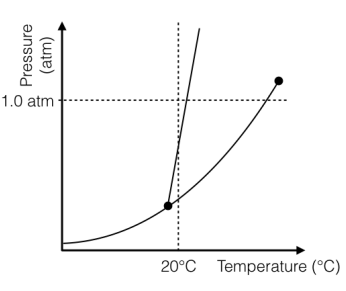Problem: The phase diagram for a substance is depicted to the below; the substance is a solid at room temperature conditions, 1.0 atm and 20°C. You wish to sublimate the solid, what change(s) must you make in order for this to occur? a) Increase the temperature. b) Decrease the pressure. c) Decrease the temperature, then decrease the pressure. d) Decrease the pressure, then increase the temperature. e) Increase the temperature, then increase the pressure.

FREE Expert Solution
79% (264 ratings)
Problem Details

The phase diagram for a substance is depicted to the below; the substance is a solid at room temperature conditions, 1.0 atm and 20°C. You wish to sublimate the solid, what change(s) must you make in order for this to occur?

a) Increase the temperature.

b) Decrease the pressure.

c) Decrease the temperature, then decrease the pressure.

d) Decrease the pressure, then increase the temperature.

e) Increase the temperature, then increase the pressure.What scientific concept do you need to know in order to solve this problem?

Our tutors have indicated that to solve this problem you will need to apply the Phase Diagrams concept. You can view video lessons to learn Phase Diagrams Or if you need more Phase Diagrams practice, you can also practice Phase Diagrams practice problems .

What is the difficulty of this problem?

Our tutors rated the difficulty of The phase diagram for a substance is depicted to the below; ... as low difficulty.

How long does this problem take to solve?

Our expert Chemistry tutor, Ellen took 4 minutes to solve this problem. You can follow their steps in the video explanation above.

What professor is this problem relevant for?

Based on our data, we think this problem is relevant for Professor Purse's class at SDSU.